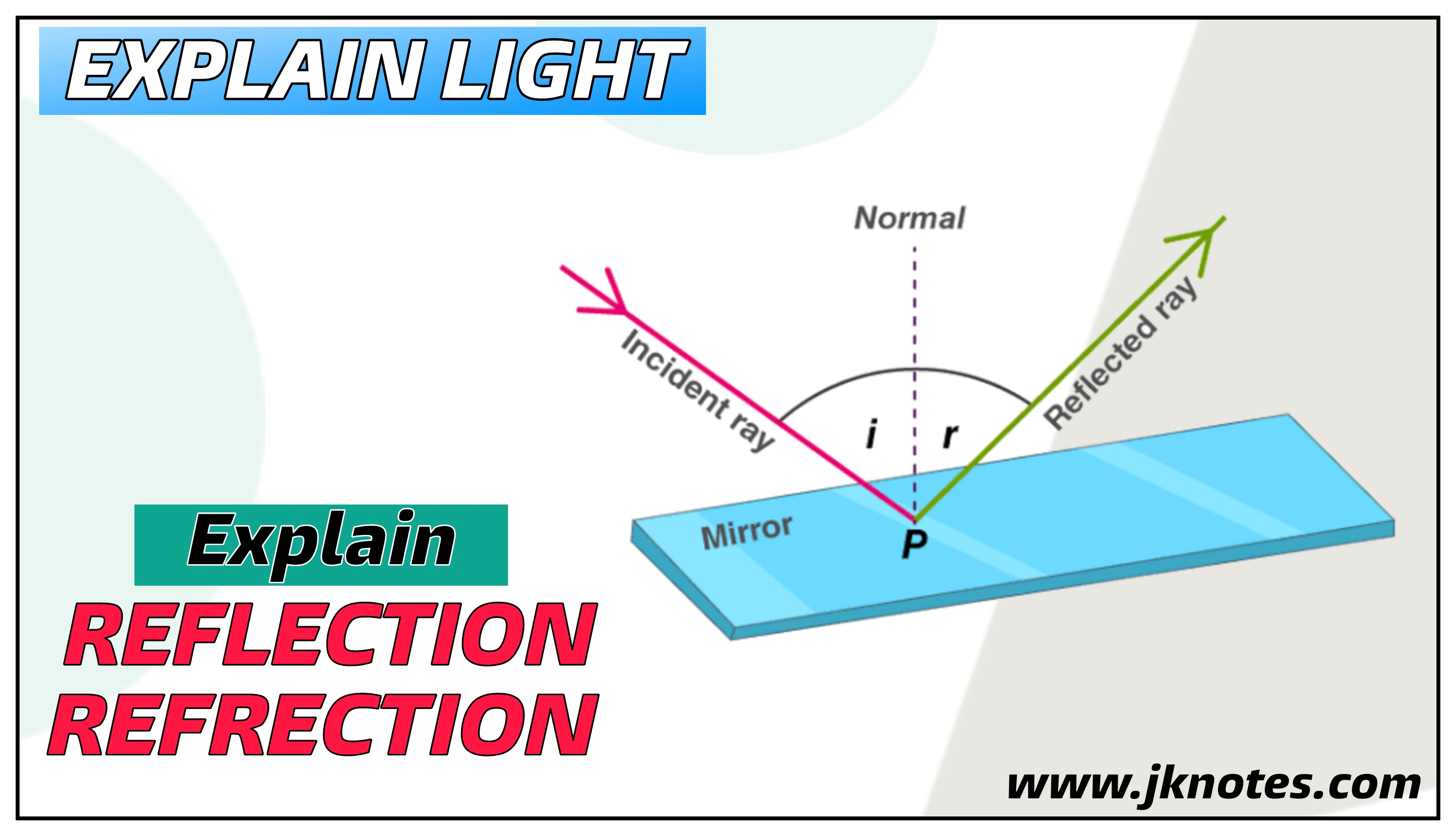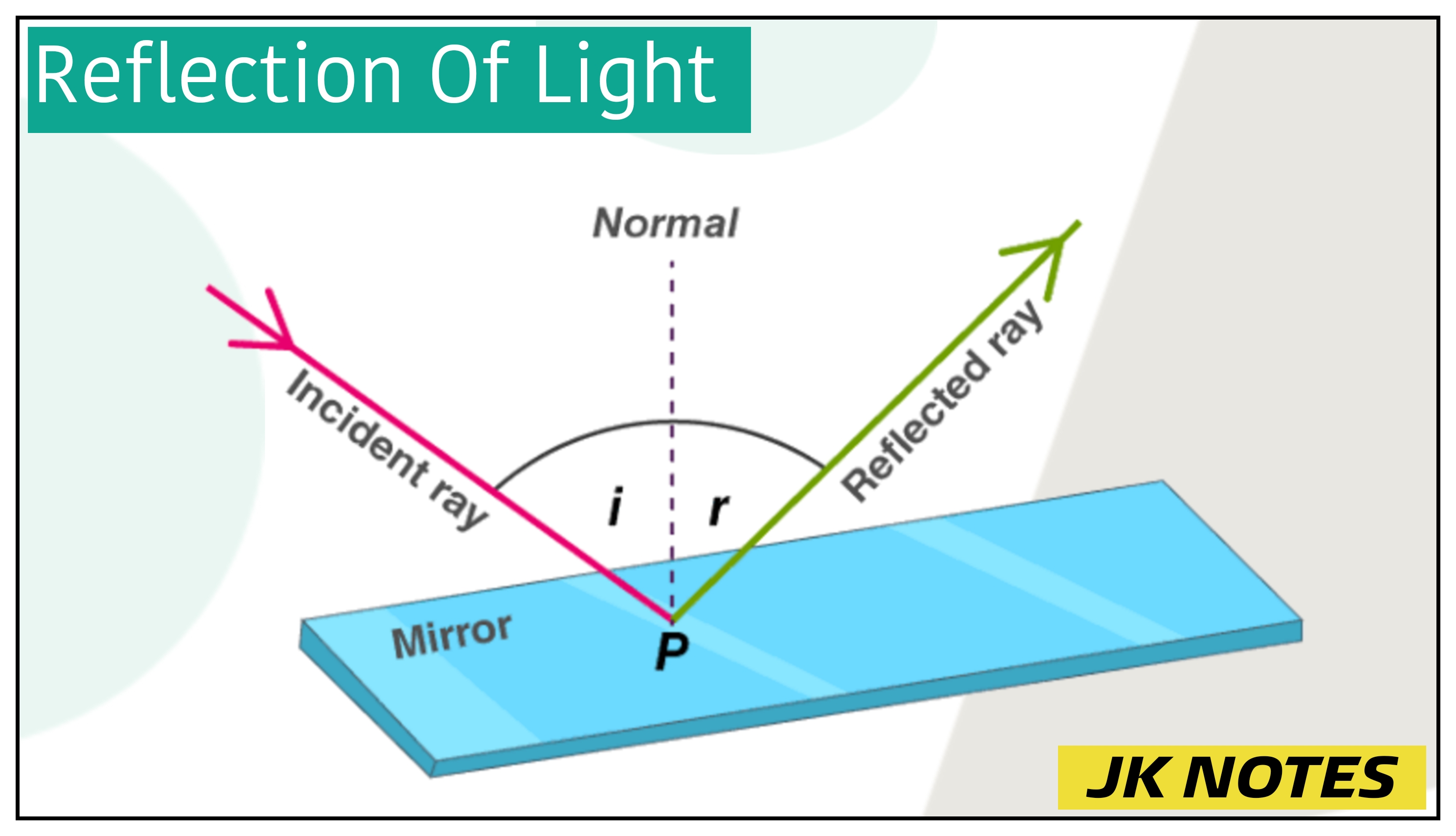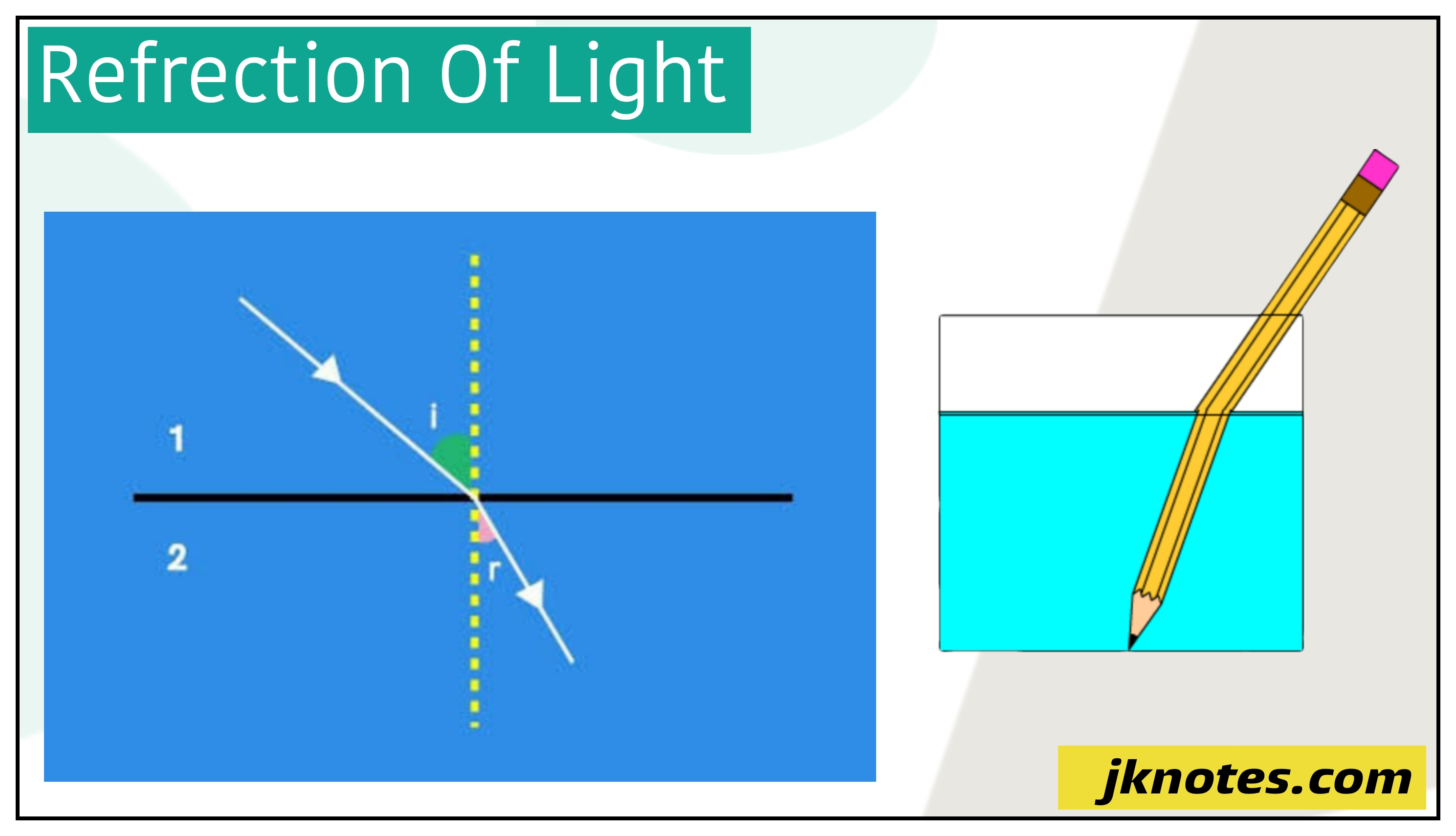# LIGHT | Explain Reflection & Refrection of Light and Explain its Laws - JK NOTES

JKBOSE-Explain Reflection & Refrection  of Light  and Explain its Laws - JK NOTESExplain Light - JKBOSE

### EXPLAIN LIGHT

Light is defined as the form of energy which  enables  us to see any objects from which it comes or from which it is reflected. Light is a form of energy which causes in us the sun sensation of sight and it always travels in straight Lines.  The fact that  small sources of light causes a  shadow in an opaque object said us that the  light travels  always in straight  line paths.

### NATURE OF LIGHT

There are two theories that explains the  nature of light:

#### Wave Theory of Light

According this theory light  consists of electromagnetic wave which has no need of material medium like solid liquid or gas for there propagation. The wavelength of visible light wave is very small but the speed of light wave is very high.

#### Particle Theory of Light

According to this theory light is consists of particles which travellers in a straight line at very high speed. The primary particle that defines  light is  Photon.

Physics experiments over the past 100 years demonstrator that light has a dual nature: light exhibits the properties of both view as and particle depends on the situation it is. In the modern theory of light called  Quantum theory of light combine is both the view and particle models of light

### REFLECTION OF LIGHTREFLECTION OF LIGHT -JKBOSE

The Reflection of light is defined as the  process of sending back of light rays that falls  on the surface of an object is known as  reflection of light. Those objects that a  having pollshed shining surface reflect more light than those object having an unpolished or dull surface. Silver metal is one of the best reflected of light. For example a silver metal  polished block reflects almost of the  all  light when  falling on it and it does not transmit any of the  light through it. But the silver metal  surface is easily scratched and it become   rough in a less amount of time . Therefore  the  ordinary mirror are consists  of  thin layer of silver metal on one side of a plane glass sheet and  than it is   protected by a coated of red paint.

#### LAWS OF REFLECTION

There are two laws of reflection which is discussed as under:

#### First Law of Reflection

It states  that the incident ray , the reflected ray and the normal all lie at the same place or they all meet at one place.

#### Second Law of Reflection

It states that the angle of incident ray is always equal to the angle of reflection .It is also defined as the angle of incident is equal to the angle of reflection. If the angle of incidence is denoted as  i and the angle of reflection is denoted as r , then the second law of reflection becomes as    < i  = < r

### REFLECTION OF LIGHTREFRECTION OF LIGHT- JKBOSE

We know that the  light travels in a straight line path. That  is true as long as light rays are travelling in the same medium having the same density throughout. If a  light rays arr going from one medium to another medium then the light rays change their direction or  it bend at the boundary between the two media. If we take an example, when a light rays travelling in 'air'  and changes its medium going into another medium like glass, then  they change their direction or they bend on the starting of  entering the glass object.

This  change in direction of light when it passes from one medium to another, is known  refraction of light. It can be defined , the bending of light when it is going  from one medium to another is known refraction of light. This refraction of light takes place when the  light rays enter from glass into air or from air into glass.

#### Laws of Refraction of Light

The refraction of light on going from one medium to another takes place according to two laws these laws  are called as the laws of refraction of light. These are given below.

#### FIrst law of refraction of light

It states that the  incident ray, the refracted ray and the normal at the point of incidence, all lie in the same plane.

#### Second law of refraction

It gives a relationship between the angle of incidence and the angle of refraction. This relationship was first discovered in 1621 by Snell experiments  so the second law of refraction is also  called Snell's law of refraction.

#### Snell's law of refraction of light

It is defined as the ratio of sine of of incidence to the sine of angle of refraction is constant for a given pair such as 'air and glass' or 'air and water'

That is :

sine of angle of incidence
---------------------          = Constant
sine of angle of refraction

OR

sin i
----         = constant
sin r

This constant is called  as refractive index

#### Effects of Refraction of Light

There are many   effects prodeced my reflection of light that  we can  easily observed in our day to day life. These effects are Under

(i) Any stick or a  pencil held obliquely and partly immersed in water appears to be bent at the water surface.

(ii) The object placed under  the water appears  raised.

(iii) A pool of water seems a less deep than the actually is.

(iv) when a thick glass slab is placed over some printed things , then when we see on the top the  letters appear raised.

# Class 10 light reflection and refraction.

### Ishaq Ramzan

Ishaq Ramzan is a Part-time blogger and Electrical Engineer. Aim to help every JK Students achieve their Goal!